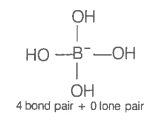The geometry of a complex species can be understood from the knowledge of the type of hybridization of orbitals of the central atom. The hybridization of orbitals of a central atom in ${\left[\mathrm{B}\left({\mathrm{OH}}_{4}\right)\right]}^{-}$ and the geometry of the complex are respectively

1. sp3, tetrahedral

2. sp3, square planar

3. sp3d2, octahedral

4. dsp2, square planar

Hint: The geometry of boron in [B(OH)4]- is tetrahedral.

Structure of ${\left[\mathrm{B}\left({\mathrm{OH}}_{4}\right)\right]}^{-}$ isHybridisation-sp3
Geometry-Tetrahedral